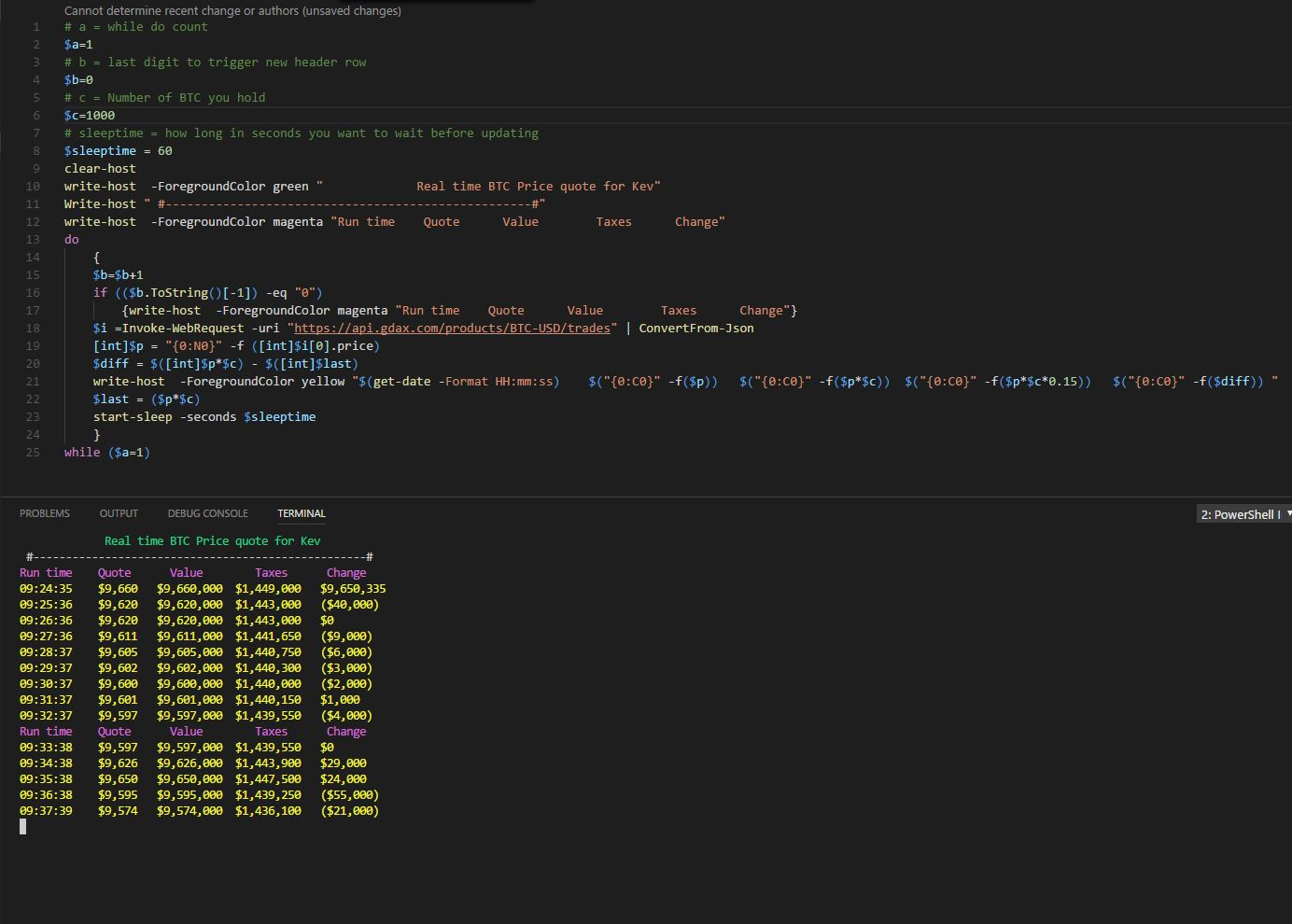My wife wrote a book - The Mystery of the Cursed Elves (The Magic Cube Book 1) -

# Run every Nth time in PowerShell

I wrote a script a while back to do an API call to GDAX to extract the current price of BTC then tell me what my holdings are worth. The script runs in a while do loop and sleeps for 600 seconds before updating the BTC quote and displaying numerical values. After a day of running the column headers are missing from the window, which bothers me. The simple answer would be to write a GUI wrapper with a scrolling window, but I am not interested in that yet.  Instead, I wanted to add headings every 10th execution.

It’s now two hours later and new headings every 10 lines is working. Turns out picking the nth digit of an integer in PowerShell is harder than I thought it would be. Grabbing the last digit of an integer is slightly simpler, but not much. When I finished I asked the team at work for alternative ideas. I think Wes gave me the simplest method for the last digit using Modulus.Script and output from Visual studio Code debug console with made up number of 1000 BTC

## Select the last digit method one – longest

PowerShell does not appear to have a simple method to select N digit in an integer, but It does have a method to select Nth character in a text string.  Before you can select the character using the string method you need to have a string to use it on. Using the string method means you have to covert your INT to a STRING then convert the results back to an INT.

In the below example I start with an integer of 12340 stored in variable \$b. Variable B is type Int32. You can see type when we hit B with .GetType() method. The next line of code converts \$b to a string, then grabs the last character [-1] = last character and shoves it into \$c. \$c is a CHAR / Character, not a string or an integer. Sadly, when we try to convert \$c directly to an Integer PowerShell, with its loose casting, converts the CHAR to its decimal ASCII representation, and not directly to an Int32 like you’d expect it to .

To get around this you can either force the casting, or convert the Char to String then convert the String to an Int32. I took the long road below with this command [int](\$c.ToString()). The forced casting way below with this command [Int]::Parse(\$c)

```PS C:\Users\kemi>\$b = 12340
PS C:\Users\kemi>\$b.GetType()
IsPublic IsSerial Name BaseType
-------- -------- ---- --------
True True Int32 System.ValueType
PS C:\Users\kemi>\$C = (\$b.ToString()[-1])
PS C:\Users\kemi>\$c.GetType()
IsPublic IsSerial Name BaseType
-------- -------- ---- --------
True True Char System.ValueType

PS C:\Users\kem>[int]\$c
48
PS C:\Users\kemi>[int](\$c.ToString())
0
PS C:\Users\kemi>[Int]::Parse(\$c)
0```

## Method two -Use Modulus Method/divide by 10

This method is super simple, but it has the drawback of only working for the last digit in a number. Which is fine for me since that is all I am after. To learn more about Modulus click link here that explains better than I could >
https://devcentral.f5.com/articles/powershell-abcs-a-is-for-arithmetic-operators

```PS C:\Users\kemi> 13932820 % 10
0
PS C:\Users\kemi> 178870 % 11
10
PS C:\Users\kemi> 1235324523451 % 10
1
PS C:\Users\kemi> 143453252 % 10
2
PS C:\Users\kemi> 12444 % 10
4
PS C:\Users\kemi> 188339 % 10
9```

Well I guess you could do some more math with Modulus to pick digits you are after like below.

```PS C:\Users\kemi> [int]\$n =12234399; ((\$n % 100) - (\$n % 10)) / 10
9
PS C:\Users\kemi> [int]\$n =12234399; ((\$n % 1000) - (\$n % 100)) / 100
3
PS C:\Users\kemi> [int]\$n =12234399; (\$n % 1000)
399
PS C:\Users\kemi> [int]\$n =12234399; (\$n % 100)
99
PS C:\Users\kemi> 399 -99
300
PS C:\Users\kemi> 300 /100
3```

## Third Method – Hard Casting

The third method involved hard casting the variable type with a tryparse. This was Ivan’s brilliant idea, and he wrote it as a new function

https://msdn.microsoft.com/en-us/library/f02979c7(v=vs.110).aspx

```function Get-LastDigitFromNumber
{
param
(
[int]\$Number
)
[int]\$result = 0;
\$stringNumber=\$Number.ToString()[-1]
if ([int]::TryParse(\$stringNumber, ([ref]\$result)))
{
return\$result;
}
return0;
}
Get-LastDigitFromNumber 10
0
Get-LastDigitFromNumber 0
0
Get-LastDigitFromNumber 11111234
4```

## Fourth Method – Splitting Method

Another method you can almost oneliner use’s -split, thanks Aaron for this one. You can split a number into an array then ask for the results -1. Minus one because when you ask for results of an array in PowerShell the array starts at 0. If you want to ask for the 9th line of an array you need to use  because of 9-1=8

`PS C:\Users\kemi> \$a = ((123456789 -split '') -ne '');\$a[((\$a.count) -5)]`
`5`